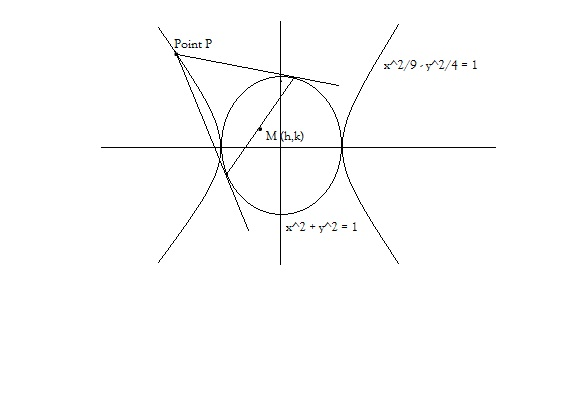# Tangents are drawn to the circle x square plus y square equal to nine from a point on the hyperbola x square by nine minus y square by four equal to one.Find the locus of the mid point of the chord of contact. Please draw the figure.

11 years ago

Hi Mayur,

Consider the point P(3sect,2tant) be any point on the hyperbola. (Refer the diagram, you'd asked for):So the equation of the chord of contact from this point is (T=0)... which is

x(3sect) + y(2tant) = 9 ----------(1)

Now the equation of the chord of contact, in terms of it's midpoint M(h.k) is (T=S1) which is,

x(h) + y(k) = h2 + k2 ----------(2)

Both (1) and (2) represent the same straight line,

So 3sect/h = 2tant/k = 9/(h2 + k2)

to find the relation interms of h,k try to eliminate t from the above relation.

So, sect = 3h/(h2 + k2) and tant = 9k/2(h2 + k2).

And we know, sec2t - tan2t = 1,

So 9h2 - (9k/2)2 = (h2 + k2)2.

And hence the locus is 9x2 - (9y/2)2 = (x2+y2)2

Hope that helps.

Best Regards,